## fullrun_ccd2_acisf12232_pi14-548_cl_pass1_csvfiles

This page was generated at Wed Feb 29 02:28:21 2012

#### Source 1

Data taken from csv/fullrun_ccd2_acisf12232_pi14-548_cl_pass1_src1.csv
X033208.66-274734.6
C/dof = -59.80/248
Total counts = 174
Model total counts = 172.5
1 const = 0
Model 1
Model 1
2 linx = 0.0009165
3 liny = 0.002364
Model 2
Model 2
4 xc = 0.07224
5 yc = 0.4178
6 norm = 7.271
7 sigmax = 0.8192 (frozen)
8 sigmay = 1.115
9 theta = 13.75
Model 3
Model 3
Model 3 Position (J2000) = 3 32 8.66 -27 47 34.6 (53.036063, -27.792935)
Model 3 Position (xc, yc) = (546.146839, 530.150771)data model resid

#### Source 2

Data taken from csv/fullrun_ccd2_acisf12232_pi14-548_cl_pass1_tfrozen_src2.csv
X033213.21-274242.2
C/dof = 207.23/354
Total counts = 38
Model total counts = 37.9
1 const = 0.02894 (0.006301 - 0.07364)
Model 1
Model 1
2 linx = 0.002261 (-0.007504 - 0.007221)
3 liny = -0.001494 (-0.008531 - 0.008515)
Model 2
Model 2
4 xc = 0.06122 (-0.6157 - 0.7832)
5 yc = -0.003835 (-0.9206 - 0.7388)
6 norm = 0.562 (0.3029 - 1.28)
7 sigmax = 1.381 (0.8141 - 2.235)
8 sigmay = 1.381 (= 7)
9 theta = 0 (frozen)
Model 3
Model 3
Model 3 Position (J2000) = 3 32 13.21 -27 42 42.2 (53.055035, -27.711715)
Model 3 Position (xc, yc) = (423.624438, 1124.507795)data model resid

#### Source 3

Data taken from csv/fullrun_ccd2_acisf12232_pi14-548_cl_pass1_src3.csv
X033201.40-274140.2
C/dof = 359.59/1591
Total counts = 45
Model total counts = 44.6
1 const = 0.01512 (0.01008 - 0.02286)
Model 1
Model 1
2 linx = -0.001401 (-0.002227 - 3.377e-05)
3 liny = -6.18e-05 (-0.0009516 - 0.001019)
Model 2
Model 2
4 xc = 0.2524 (-0.6264 - 1.07)
5 yc = -0.1374 (-1.138 - 0.9151)
6 norm = 0.3448 (0.1954 - 0.5616)
7 sigmax = 1.5 (>0.8766)
8 sigmay = 1.483 (>1.46)
9 theta = -251.1 (indef)
Model 3
Model 3
Model 3 Position (J2000) = 3 32 1.40 -27 41 40.2 (53.005824, -27.694498)
Model 3 Position (xc, yc) = (742.513022, 1250.279301)data model resid

#### Source 4

Data taken from csv/fullrun_ccd2_acisf12232_pi14-548_cl_pass1_tfrozen_src4.csv
X033210.03-274303.5
C/dof = 213.86/434
Total counts = 30
Model total counts = 31.3
1 const = 0.07574 (0.0523 - 0.09995)
Model 1
Model 1
2 linx = 0.01633 (0.004222 - 0.02601)
3 liny = -0.003358 (-0.00997 - 0.005277)
Model 2
Model 2
4 xc = 6.387 (>5.589)
5 yc = -6.254 (indef)
6 norm = 1.32e-06 (<0.0006573)
7 sigmax = 0.7136 (indef)
8 sigmay = 0.7136 (= 7)
9 theta = 0 (frozen)
Model 3
Model 3
Model 3 Position (J2000) = 3 32 10.03 -27 43 3.5 (53.041777, -27.717627)
Model 3 Position (xc, yc) = (509.481646, 1081.211118)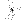data model resid

#### Source 5

Data taken from csv/fullrun_ccd2_acisf12232_pi14-548_cl_pass1_tfrozen_src5.csv
X033210.87-274415.2
C/dof = 108.57/249
Total counts = 25
Model total counts = 25.4
1 const = 0 (<0.02524)
Model 1
Model 1
2 linx = -0.004986 (-0.01986 - 0.002945)
3 liny = 0.003437 (-0.004935 - 0.01792)
Model 2
Model 2
4 xc = 0.3149 (-0.1172 - 0.7683)
5 yc = 0.2596 (-0.1446 - 0.6836)
6 norm = 1.001 (0.5511 - 1.686)
7 sigmax = 0.9775 (0.84 - 1.268)
8 sigmay = 0.9775 (= 7)
9 theta = 0 (frozen)
Model 3
Model 3
Model 3 Position (J2000) = 3 32 10.87 -27 44 15.2 (53.045291, -27.737546)
Model 3 Position (xc, yc) = (486.640083, 935.472415)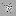data model resid

#### Source 6

Data taken from csv/fullrun_ccd2_acisf12232_pi14-548_cl_pass1_tfrozen_src6.csv
X033202.34-274338.0
C/dof = 233.43/777
Total counts = 27
Model total counts = 28.0
1 const = 0.0373 (0.02327 - 0.05178)
Model 1
Model 1
2 linx = 0.002928 (-0.002712 - 0.00627)
3 liny = -0.003366 (-0.00664 - 0.002796)
Model 2
Model 2
4 xc = -9.397 (0 - -9.397)
5 yc = 9.397 (>8.428)
6 norm = 1e-06 (<0.0005034)
7 sigmax = 4.699 (indef)
8 sigmay = 4.699 (= 7)
9 theta = 0 (frozen)
Model 3
Model 3
Model 3 Position (J2000) = 3 32 2.34 -27 43 38.0 (53.009746, -27.727216)
Model 3 Position (xc, yc) = (716.899939, 1010.899939)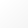data model resid

#### Source 7

Data taken from csv/fullrun_ccd2_acisf12232_pi14-548_cl_pass1_tfrozen_src7.csv
X033148.32-274306.6
C/dof = 402.97/2394
Total counts = 40
Model total counts = 40.3
1 const = 0.01667 (0.01224 - 0.02264)
Model 1
Model 1
2 linx = -0.0009102 (-0.001663 - 0.0002913)
3 liny = -0.000535 (-0.001225 - 0.0005145)
Model 2
Model 2
4 xc = 16.14 (>14.55)
5 yc = -16.14 (indef)
6 norm = 1e-06 (<0.001009)
7 sigmax = 1.009 (indef)
8 sigmay = 1.009 (= 7)
9 theta = 0 (frozen)
Model 3
Model 3
Model 3 Position (J2000) = 3 31 48.32 -27 43 6.6 (52.951334, -27.718493)
Model 3 Position (xc, yc) = (1095.314431, 1074.314431)data model resid

#### Source 8

Data taken from csv/fullrun_ccd2_acisf12232_pi14-548_cl_pass1_tfrozen_src8.csv
X033201.38-274648.6
C/dof = 128.64/249
Total counts = 25
Model total counts = 22.4
Warning, poor agreement between model and actual number of counts may be indicative of a poor fit
1 const = 0 (<0.0407)
Model 1
Model 1
2 linx = 0.01312 (-0.007616 - 0.04077)
3 liny = -0.01722 (-0.03988 - -0.004849)
Model 2
Model 2
4 xc = 0.2176 (-0.4849 - 0.8421)
5 yc = 0.3209 (-0.2262 - 0.9633)
6 norm = 0.5996 (0.2945 - 1.057)
7 sigmax = 1.254 (0.8751 - 1.686)
8 sigmay = 1.254 (= 7)
9 theta = 0 (frozen)
Model 3
Model 3
Model 3 Position (J2000) = 3 32 1.38 -27 46 48.6 (53.005750, -27.780178)
Model 3 Position (xc, yc) = (742.442331, 623.347799)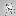data model resid

#### Source 9

Data taken from csv/fullrun_ccd2_acisf12232_pi14-548_cl_pass1_tfrozen_src9.csv
X033200.68-274324.2
C/dof = 120.20/249
Total counts = 16
Model total counts = 17.4
1 const = 0.07247 (0.0418 - 0.1066)
Model 1
Model 1
2 linx = 0.01057 (-0.01224 - 0.02198)
3 liny = -0.007373 (-0.01924 - 0.008126)
Model 2
Model 2
4 xc = -4.511 (indef)
5 yc = 4.511 (>4.112)
6 norm = 1e-06 (<1.799)
7 sigmax = 0.6841 (indef)
8 sigmay = 0.6841 (= 7)
9 theta = 0 (frozen)
Model 3
Model 3
Model 3 Position (J2000) = 3 32 0.68 -27 43 24.2 (53.002813, -27.723393)
Model 3 Position (xc, yc) = (761.830894, 1038.830894)data model resid

#### Source 10

Data taken from csv/fullrun_ccd2_acisf12232_pi14-548_cl_pass1_tfrozen_src10.csv
X033152.46-274640.3
C/dof = 206.12/1082
Total counts = 24
Model total counts = 23.7
1 const = 0.01096 (0.005051 - 0.02041)
Model 1
Model 1
2 linx = 0.0002767 (-0.001269 - 0.001427)
3 liny = 0.000175 (-0.001233 - 0.001727)
Model 2
Model 2
4 xc = 1.245 (0.06203 - 2.597)
5 yc = 0.2539 (-1.286 - 1.427)
6 norm = 0.1752 (0.07664 - 0.4215)
7 sigmax = 1.608 (0.6768 - 2.538)
8 sigmay = 1.608 (= 7)
9 theta = 0 (frozen)
Model 3
Model 3
Model 3 Position (J2000) = 3 31 52.46 -27 46 40.3 (52.968590, -27.777871)
Model 3 Position (xc, yc) = (983.030447, 639.983990)data model resid

#### Source 11

Data taken from csv/fullrun_ccd2_acisf12232_pi14-548_cl_pass1_tfrozen_src11.csv
X033209.42-274807.2
C/dof = 85.95/249
Total counts = 16
Model total counts = 13.2
Warning, poor agreement between model and actual number of counts may be indicative of a poor fit
1 const = 0.00154 (<0.02127)
Model 1
Model 1
2 linx = -0.01471 (-0.02125 - -0.003059)
3 liny = 0.01337 (-0.001454 - 0.02839)
Model 2
Model 2
4 xc = 0.2859 (-0.1144 - 0.4861)
5 yc = 0.5626 (0.1505 - 0.7687)
6 norm = 1.417 (0.7667 - 2.25)
7 sigmax = 0.5484 (0.4252 - 0.6946)
8 sigmay = 0.5484 (= 7)
9 theta = 0 (frozen)
Model 3
Model 3
Model 3 Position (J2000) = 3 32 9.42 -27 48 7.2 (53.039234, -27.801997)
Model 3 Position (xc, yc) = (525.581065, 463.856543)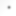data model resid